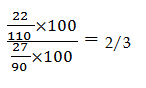# RRB ALP 2018 Practice Test Papers | Arithmetic Questions (Day-69)

Dear Aspirants, Here we have given the Important RRB ALP & Technicians Exam 2018 Practice Test Papers. Candidates those who are preparing for RRB ALP 2018 can practice these Arithmetic Questions to get more confidence to Crack RRB 2018 Examination.

[WpProQuiz 2959]

Click “Start Quiz” to attend these Questions and view Solutions

1) Find the profit or loss percentage of a transaction of Amitif he bought a painting for Rs. 1000 and sold it Rs.1500.

a) 150% profit

b) 50 % profit

c) 100% loss

d) 25% loss

2) Find the value of x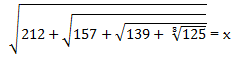a) 19

b) 17

c) 15

d) 12

3) A Women can do a piece of work in 6 days. Men can do that work in 4 days. If a man, a women and a boy can complete that work in 2 days. In How many days the work will be finished if theboy alone do that work?

a) 15 days

b) 12 days

c) 10 days

d) 8 days

4) Find the value of x

529÷x = (2^2×5+3)

a) 37

b) 29

c) 23

d) 19

5) A boat can row upstream in 20 km in 5 hours and downstream in 4 hours. What is the Ratio of Speed of stream and boat of speed?

a) 1: 9

b) 1: 8

c) 1: 7

d) 1:6

6) 12% of 40 + 15% of 78 = x+ 6.5

a) 12

b) 14

c) 15

d) 10

7) Find the Sum of Money for which the difference between Simple Interest and Compound Interest obtained on it in 2 years at 4 % per annum compounded annually is Rs. 50

a) 35000

b) 62500

c) 31250

d) 25310

8) The ratio of Length, Width and Height ofa  Cuboid is 4:5:6 respectively. Find the Value of Height if Volume of cuboid is 3240.

a) 12

b) 18

c) 30

d) 24

9) What is the value ofSin2θ?

a) 2sinθ cos θ

b) cos2 θ

c) sin2 θ. Cos2 θ

d) sin2 θ

10)The numerator is increased by 10% and the denominator value decreased by 90 the new fraction is 22/27. Find the original fraction.

a) 3/4

b) 3/7

c) 2/3

d) 2/5

Cost Price = 1000

Selling Price = 1500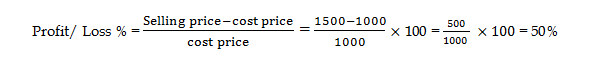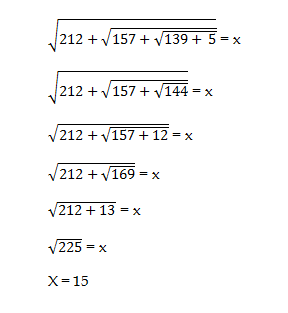Women – 1/ 6 days

Men – 1/4 days

Women + Men + Boy = ½ days

1/6 + 1/ 4 + Boy = ½ days

5/12 + boy = ½

Boy = 1/2 – 5/ 12 = 6/ 12 – 5/12 = 1/ 12

Boy can complete the work in 12 days

529÷ x = (4×5+3) = 23

529 = 23x

X = 529÷23

X = 23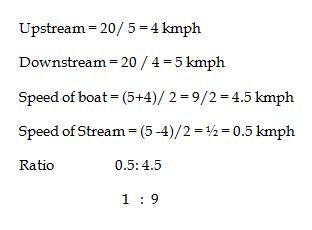12% of 40 + 15% of 78 = x+ 6.5

4.8 + 11.7 = x + 6.5

16.5 + x =6.5

X =10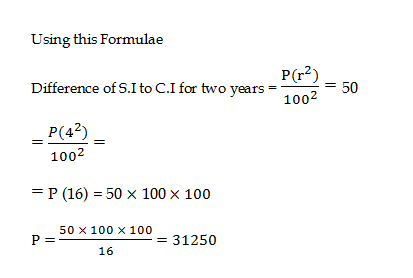Volume of Cuboid = lbh

Ratio of lbh = 4:5:6

4x*5x*6x = 3240

120x3 = 3240

X3 = 27

X = 3

L = 12 B = 15             H =18

Sin2 θ = 2sinθcos θ